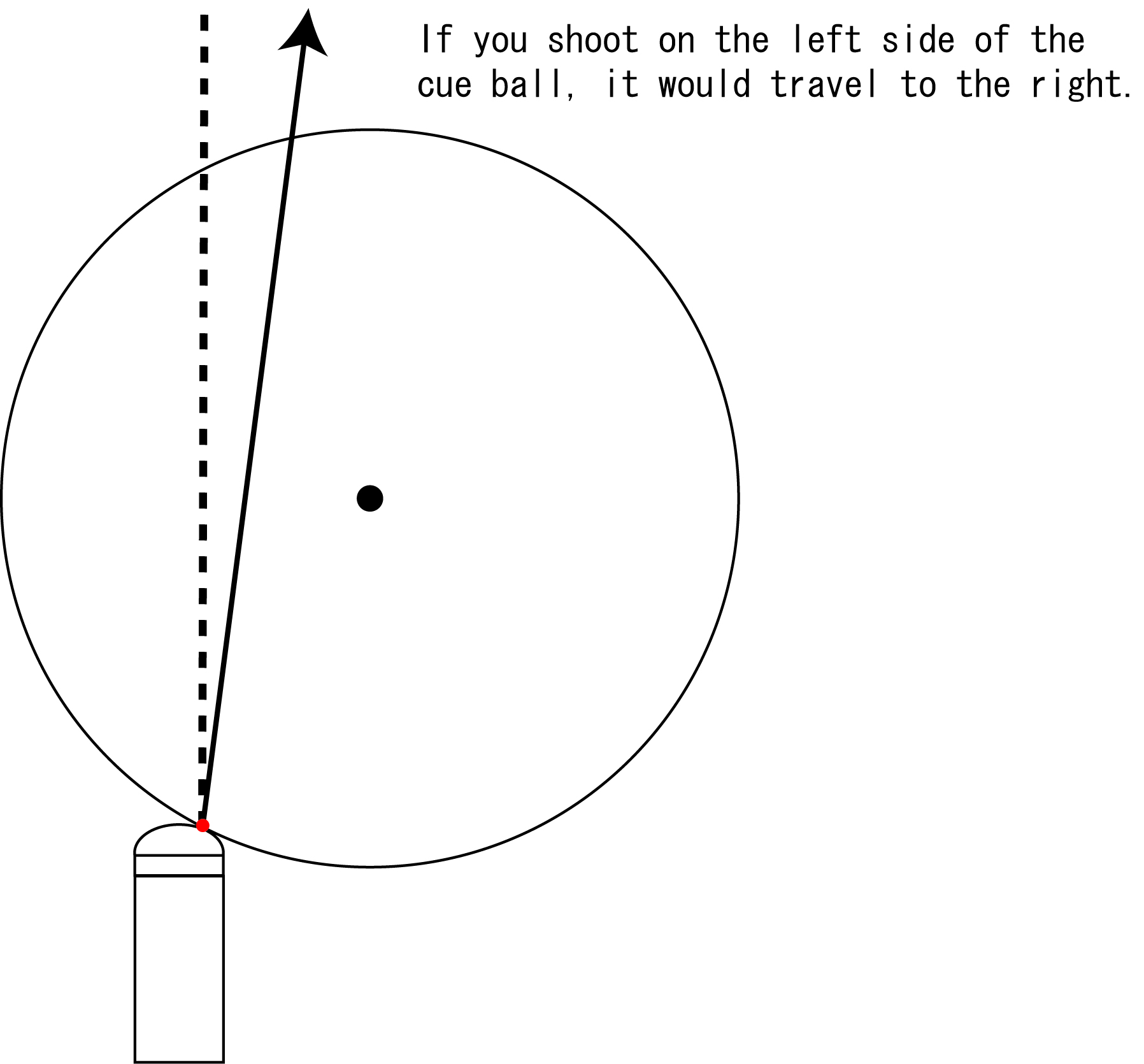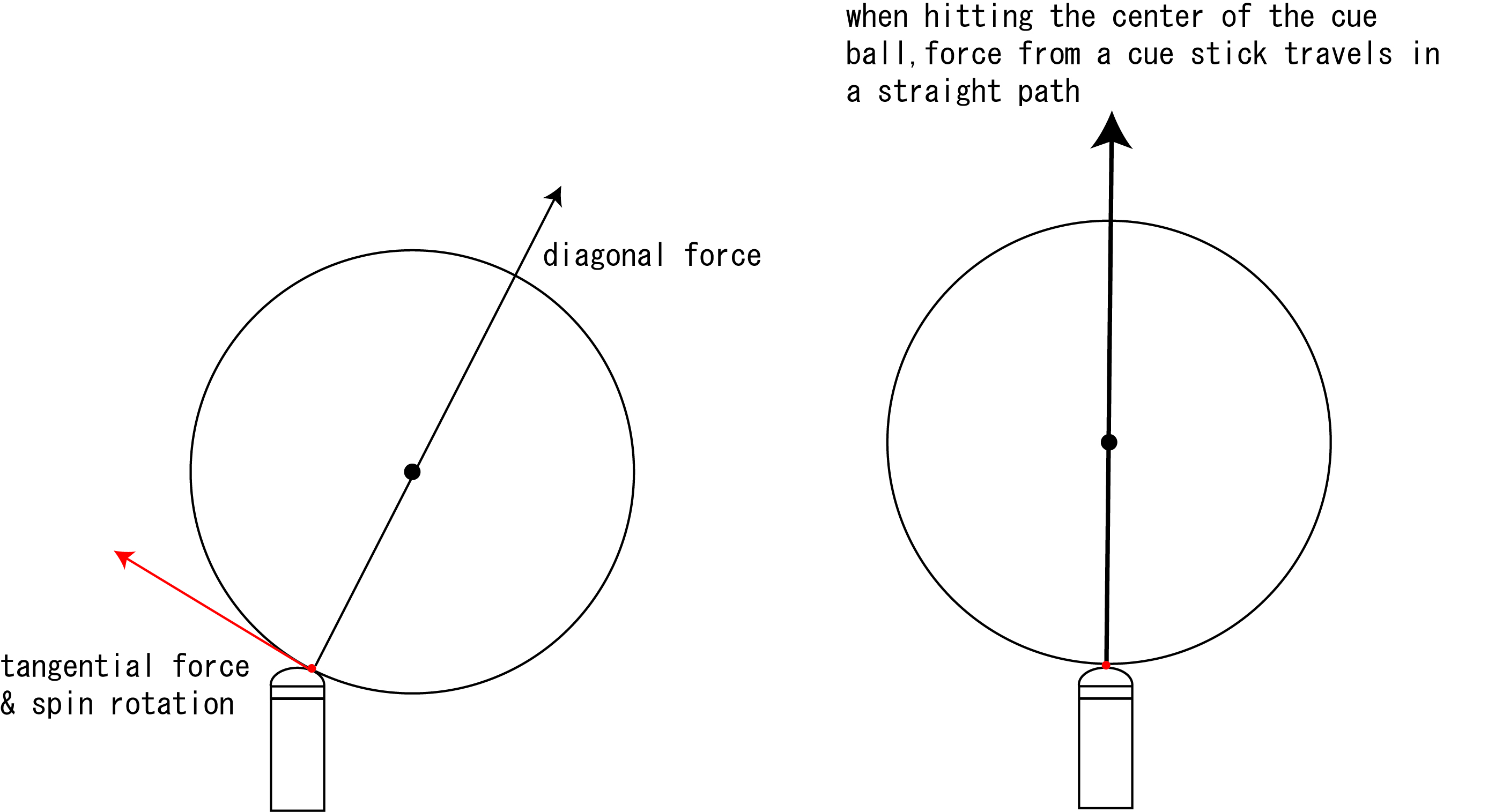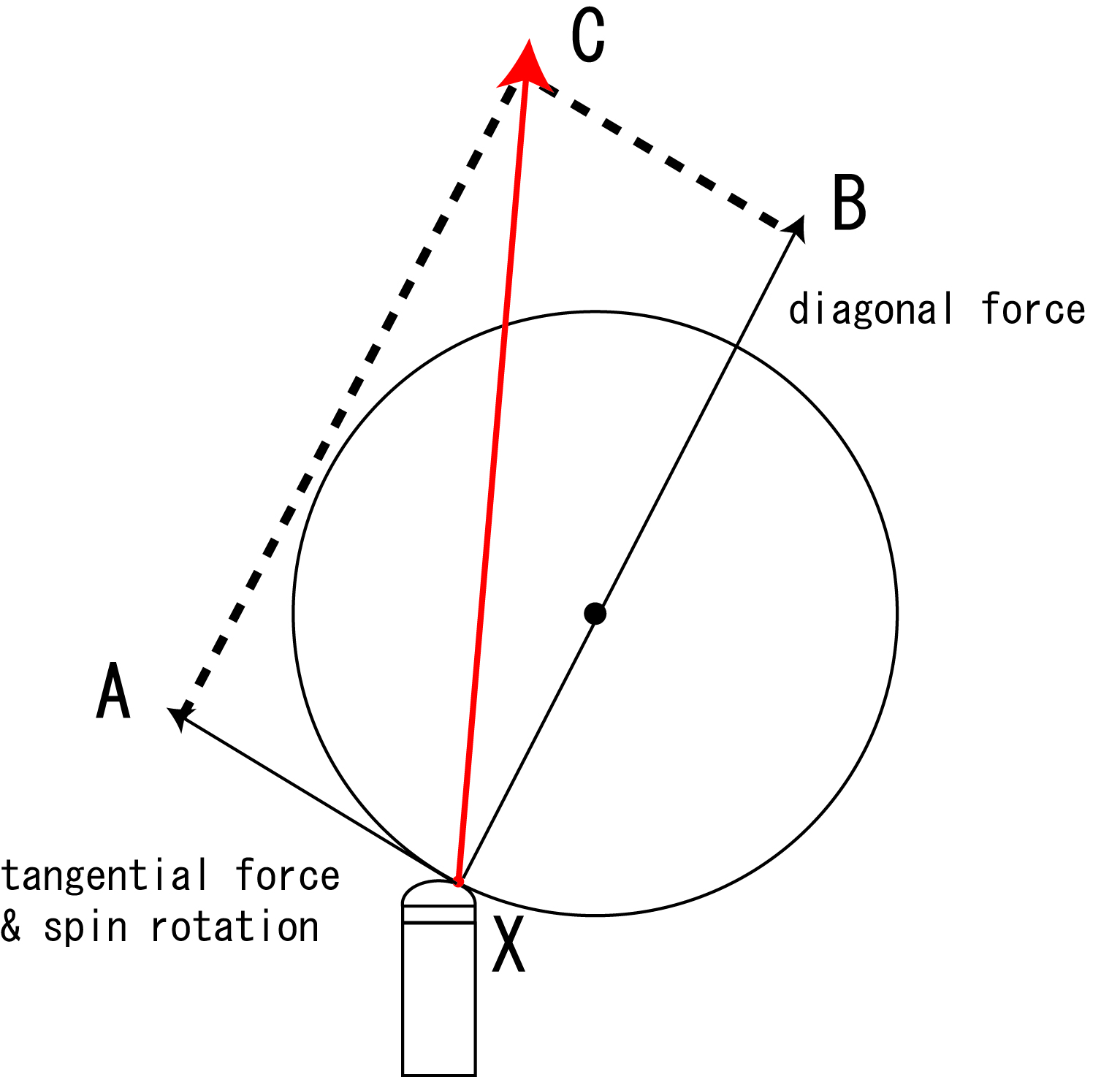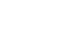# Understanding cue ball deflection

In this chapter, I would explain the reason why cue ball deflection happens. To keep the explanation brief, the frictional force between the cue ball and cloth is not considered.

Step1: Before everything, please understand that when you shoot the left side of the cue ball, the cue ball would deflect and go the right direction. Imagine when you try to move an object ball to right side, the cue ball has to be hit on the left direction of the object ball. This is the basic mechanism of cue ball deflection.Why does the cue ball deflect?

Step2:
When you apply English on the cue ball, the cue force is divided into diagonal movement that is directed to the center of the cue ball and tangential force & spin rotation.The reason why cue ball deflects, when hitting the cue ball with side English, the force of the cue ball is divided into a diagonal direction and tangential direction. From a physics point of view, the cue ball travels to a direction that comes from the force of vectorX-A and X-B as the picture below shows. This is how cue ball deflection is calculated.If we deeply understand why deflection is caused, we are able to reduce it. In the case of picture above, reducing vectorX-B and increasing vectorX-A bring about less deflection.

This picture shows how deflection is decreased. Increasing vectorX-A and reducing vectorX-B changes cue ball direction that is dependant of the resulting force. Cue ball path shifts direction X-C to X-D when vector X-A is increased.It is a common way to use low deflection shafts to reduce deflection. Furthermore, KAMUI chalk also can reduce it. I would explain how KAMUI chalk works to reduce deflection on ‘KAMUI chalk reduces deflection’ page.